# Ohba's Conjecture (Solved)

 Importance: Medium ✭✭
 Author(s): Ohba, Kyoji
 Subject: Graph Theory » Coloring » » Vertex coloring
 Keywords: choosability chromatic number complete multipartite graph list coloring
 Posted by: Jon Noel on: May 18th, 2011
 Solved by: Noel, Reed and Wu. Submitted.
Conjecture   If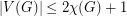, then.

## Basics of List Colouring

A-list assignment of a graphis an assignment of listsof`available colours' to the vertices of. A graph is said to be-choosable if it can be properly coloured from the prescribed lists, and-choosable if this property holds for every-list assignment.

Definition   Given a graph, we define the list chromatic number ofby.

The lower boundis easily observed by assigning the same list to every vertex. However, the reverse inequality does not hold in general. In their classic paper , Erdos, Rubin and Taylor give the example of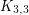where the three vertices on either side of the bipartition are given lists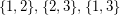. One may check that no list colouring exists despite the fact that all lists have size two. Note that this example shows that the conjecture, if true, would be tight.

In general, there are bipartite graphs with arbitrarily high list chromatic number and socan be arbitrarily large. A natural question arises: What conditions guarantee that? A graphwith this property is said to be chromatic-choosable.

## The Conjecture

Erdos, Rubin and Taylor proved that every multipartite graph with all parts of size two is chromatic-choosable . In  Gravier and Maffray show that the propertiy still holds if one of the parts has size three. Ohba's Conjecture  above can be seen as a generalization. Some weaker versions have been proven:

Theorem  (Ohba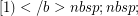] If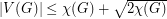, thenis chromatic-choosable.
Theorem  (Reed and Sudakov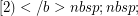] If, thenis chromatic-choosable.
Theorem  (Reed and Sudakov] Ifwhereand, thenis chromatic-choosable.

One may observe that Ohba's conjecture holds for all graphs if and only if it holds for complete multipartite graphs. Indeed, given a counterexample we may add an edge between vertices of different colour classes to yeilds another counterexample. Thus, the main focus of [6-11] has been on verifying Ohba's conjecture for certain classes of multipartite graphs. It is now known that complete multipartite graphs having parts of size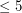satisfy Ohba's conjecture . In other words, the conjecture is solved for graphs with independance number at most five.

Ohba's Conjecture can be found as unsolved problem #50 in Bondy and Murty's book "Graph Theory" .

The problem was recently solved . The proof can be found here. It can also be found here. Click here for a problem which generalizes Ohba's Conjecture.

## Bibliography

* K. Ohba. On chromatic-choosable graphs, J. Graph Theory. 40 (2002) 130--135. MathSciNet.

 B. Reed, B. Sudakov. List colouring when the chromatic number is close to the order of the graph, Combinatorica 25 (1) (2004) 117--123. MathSciNet.

 B. Reed, B. Sudakov. List colouring of graphs with at mostvertices, Beijing, 2002, in: Proceedings of the International Congress of Mathematicians, vol. III, Higher Ed. Press, Beijing (2002) 587–-603.

 P. Erdős, A. L. Rubin, H. Taylor, Choosability in graphs, Proc. West Coast Conference on Combinatorics, Graph Theory and Computing, Arcata, Congressus Numerantium 26 (1979) 125–-157. pdf.

 H. Enomoto, K. Ohba, K. Ota, J. Sakamoto. Choice number of some complete multi-partite graphs, Discrete Math. 244 (1-3) (2002) 55--66.

 K. Ohba. Choice number of complete multipartite graphs with part size at most three, Ars Combin. 72 (2004) 133--139.

 Y. Shen, W. He, G. Zheng, Y. Wang, L. Zhang. On choosability of some complete multipartite graphs and Ohba's conjecture, Discrete Math. 308 (1) (2008) 136--143.

 W. He, L. Zhang, D. W. Cranston, Y. Shen, G. Zheng. Choice number of complete multipartite graphs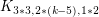and, Discrete Math. 308 (23) (2008) 5871–-5877.

 G. Zheng, Y. Shen, Z. Chen, J. Lv. On choosability of complete multipartite graphs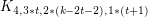, Discuss. Math. Graph Theory 30 (2) (2010) 237–-244.

 A. V. Kostochka, M. Stiebitz, D. R. Woodall. Ohba's conjecture for graphs with independence number five, Discrete Math. 311 (12) (2011) 996--1005. ScienceDirect.

 S. Gravier, F. Maffray. Graphs whose choice number is equal to their chromatic number, J. Graph Theory 27 (1998) 87--97. MathSciNet.

 J. A. Noel, B. A. Reed, H. Wu. A Proof of a Conjecture of Ohba. Submitted. pdf.

 J. A. Noel. Choosability of Graphs with Bounded Order: Ohba's Conjecture and Beyond. Master's Thesis, McGill University. pdf.

 J. A. Bondy and U. S. R. Murty. Graph theory, volume 244 of Graduate Texts in Mathematics. Springer, New York, 2008.

* indicates original appearance(s) of problem.